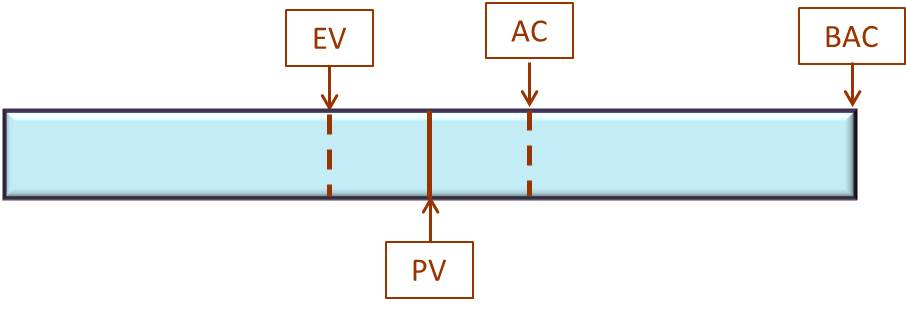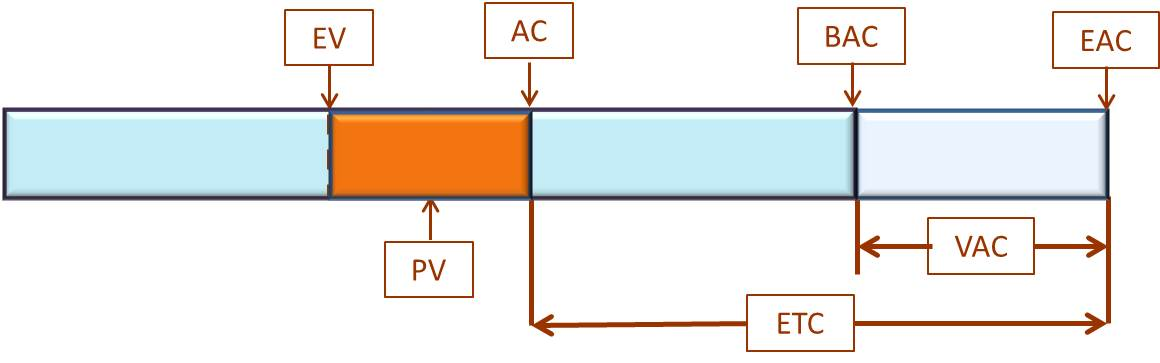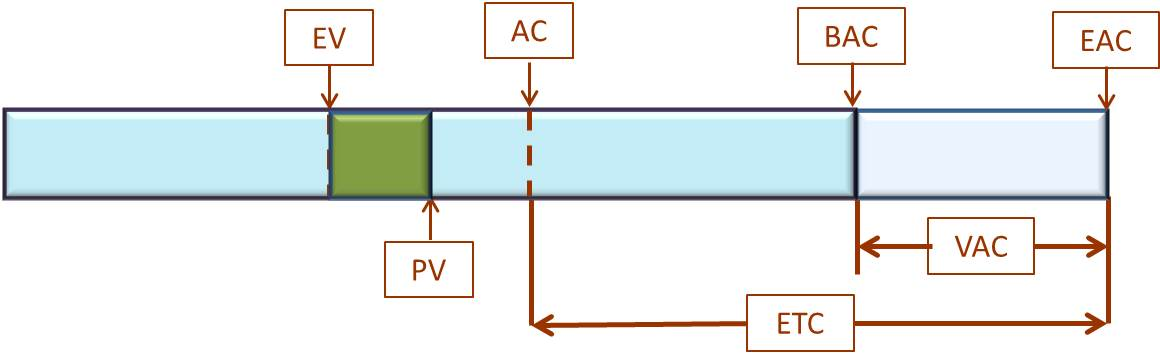# Understanding Earned Value Management: Part 3 – Earned Value Mathematics Project’s Current State

### PMBOK®Guide Definition:

Cost Variance (CV): The amount of budget deficit or surplus at a given point in time, expressed as the difference between the earned value and the actual cost.

Schedule Variance (SV): A measure of schedule performance expressed as the difference between the earned value and the planned value.

Cost Performance Index (CPI): A measure of cost efficiency of budgeted resources expressed as the ratio of earned value to actual cost.

Schedule Performance Index (SPI): A measure of schedule efficiency expressed as the ratio of earned value to planned value.

### Practical Definition:

Cost Variance (CV): The difference between project progress (earned value) and the actual cost (actual cost) at a given point in the schedule.

Schedule Variance (SV): The difference between project progress (earned value) and the planned progress (planned value) at a given point in the schedule.

Cost Performance Index (CPI): Measures our efficiency of money spent on the project. Are we getting a dollar of progress for every dollar spent?

Schedule Performance Index (SPI): Measures our efficiency of work on the project. For every hour worked, are we getting one hour of progress?

Note: This is the third article in a series describing Earned Value Management (EVM). The first article uses a conceptual model describing the current state of a project. The second article uses a conceptual model describing the forecasting of the project. This article defines the mathematics behind the current project state conceptual model of the first article. The fourth article puts the mathematics behind the second article’s conceptual model for forecasting the project. The fifth article provides the bigger picture of Earned Value Management within the context of the overall project.

### Current State Formulas

Building on the conceptual model of the first two articles in this series, let’s puts some equations to the diagrams.Conceptual Model of a Project’s Earned Value Management

Quick refresher on the use of our variables:

• Earned Value (EV) defines the progress made on the project (about 40% in diagram).
• Planned Value (PV) shows the time spent on the project (about 50%).
• Actual Cost (AC) indicates the amount we’ve spent so far (60%).
• Budget at Completion (BAC) is the budget for the project – in our case, \$10,000.

Let’s translate those percentages into values:

• EV = \$4,000 (40% of \$10,000)
• PV = \$5,000 (50% of \$10,000)
• AC = \$6,000 (60% of \$10,000)

Four values define the current state of the project:

• Cost Variance (CV) – difference between what we’ve spent and the progress made,
• Schedule Variance (SV) – difference between the current progress and the planned progress at this point in the project,
• Cost Performance Index (CPI) – are we getting full value for the money spent on the project, and
• Schedule Performance Index (SPI) – are we getting full value for the time spent working on the project?

### Project’s Current Status Equations

A typical stumbling block for those studying for the Project Management Professional (PMP®) exam is memorizing the equations. Here are two tricks to memorizing these equations:

1. Write them down in the same order each time, and
2. Remember these simple hints to memorizing the formulas.

The equations consist of the three main variables: EV, PV and AC. Here are the equations:

• CV = EV – AC
• SV = EV – PV
• CPI = EV / AC
• SPI = EV / PV

Since we write these equations in the same order, we know the second hint for writing them correctly:

• First, we simply list each variable – CV, SV, CPI, and SPI.
• Next to them, we write the equals sign (=).
• Next, note each equation starts with EV.
• We know variances are expressed as differences, i.e., the subtraction operator (-). Indexes are simply ratios which are expressed as division (/).

Here’s the tricky part. The final variable, AC or PV, determines the resulting values. Cost variance and Cost Performance Index has to do with cost, therefore, the only logical variable used would be AC. The remaining variable is PV – process of elimination. Simply write AC and PV at the end of the respective equation.  With that hint, you can almost noodle your way through remembering the equations.

Using our values for EV, AC, and PV from above, we can solve these equations:

• CV = \$4,000 (EV) – \$6,000 (AC) = -\$2,000
• SV = \$4,000 (EV) – \$5,000 (PV) = -\$1,000
• CPI = \$4,000 (EV) / \$6,000 (AC) = .667
• SPI = \$4,000 (EV) / \$5,000 (PV) = .8

### Understanding the Values

#### Cost Variance

Cost variance provides the difference between what we budgeted versus what we’ve actually spent. Naturally, if cost variance is negative, we’ve over spent.  We spent more money than we had planned to spend.  We call that over-budget. If cost variance is positive, we’ve spent less money than we expected, a.k.a., under-budget. Cost variance is depicted below – the difference between EV and AC.Cost Variance – difference between AC and EV

#### Schedule Variance

Schedule variance indicates whether we are getting the value from the hours worked. It is expressed in terms of money. If we’ve spent 5 hours working on a task, do we have 5 hours of progress made on the task? If no, how many hours will it take to get to the progress level planned? Represented in money, we would simply multiply the hourly rate and hours remaining giving us the schedule variance. If schedule variance is negative, we are behind schedule – we are not getting the full value of the time worked. If schedule variance is positive, we are efficient and getting more value for our efforts than originally planned, a.k.a., ahead of schedule.

Schedule variance is depicted below – the difference between EV and PV.Schedule Variance – difference between PV and EV

Cost variance and schedule variance of zero (0) mean on-budget and on-time. The rule for the variances is:

• Variance >= 0   Good

The variances represent the differences but not our efficiency. The indexes indicate our efficiency.

### Cost Performance Index and Schedule Performance Index

Cost Performance Index indicates how efficiently we are spending our money. In our example above, CPI equals .667. Indexes less than one (1) indicate a negative efficiency. An index greater than one (1) indicates positive efficiency.   The further away from one (1) indicates a more negative or positive stance.

In our example, CPI being .667, we are in pretty bad shape. We will need to take some drastic measures to recover the budget. The Schedule Performance Index (.8) is negative also, but not as bad as the Cost Performance Index. If we could only focus on one aspect of the project, we would focus on spending rate.

The rule for the Performance Indexes is

• Performance Index < 1      Bad
• Performance Index >= 1   Good

### Conclusion

In this article, we described the equations used to understand the current state of a project. These equations give us great insight into the project’s current health and show us where we are excelling or missing the mark. The variances provide the values by which we differ from our plan while the indexes show our project performance.

In the next article, we describe the forecasting formulas and what we need to do in order to bring our project in line with the plan.